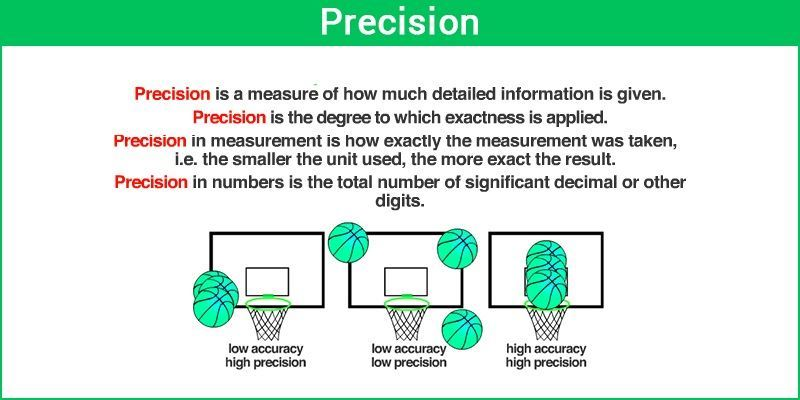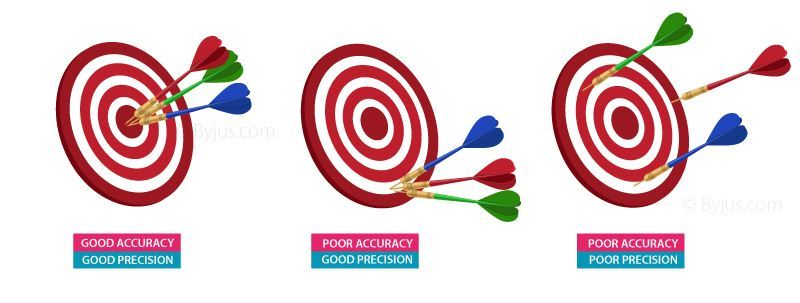# Precision

Precision refers to the amount of information that is conveyed by a number in terms of its digits; it shows the closeness of two or more measurements to each other. It is independent of accuracy.

## Precision Definition

If you weigh an object five times and you get 3.2 kg every time, then your measurement is very precise. Precision refers to a value in decimal numbers after the whole number, and it does not relate to accuracy. The concepts of accuracy and precision are almost related, and it is easy to get confused.Precision is a number that shows an amount of the information digits and it expresses the value of the number.
For Example- The appropriate value of pi is 3.14 and its accurate approximation. But the precision digit is 3.199 which is less than the exact digit. It has numerous forms in statistics, arithmetic, precision etc.

## Difference Between Precision and Accuracy

For instance:

• A number that is not precise but accurate.
• A number that is not accurate but precise.
• A number that is precise and accurate.

Precision is the amount of information that is conveyed by a value. Whereas Accuracy is the measure of correctness of the value in correlation with the information.

Let’s consider the value of “pi”, i.e, 3.142857143.

According to the questions:

A number that is not precise but accurate is 3.14. It is accurate based on closeness and no other number with three digits can get closer to the target.

A number that is not accurate but precise is 1.1423345678901234567890. It is very precise as it conveys more information. But cannot be considered accurate because it’s not near the target.

A number that is precise and accurate is 3.142857143. It is the only number which has maximum accuracy and precision if the target value is 3.142857143.The above figure shows a dart game having three images,

(i) The first one shows Good accuracy and good precision, as all the three darts are close to the maximum score region (accurate) and also all the darts are close to each other (precise).

(ii) The second figure shows Poor accuracy but good precision, as all the three darts are close enough but far away from the maximum score region.

(iii) The third figure shows Poor accuracy and poor precision, as all the darts are neither nearby nor to the maximum score region.

### Precision and Recall

In pattern identification, data retrieval and analysis, precision or the positive predictive value is the fraction of relevant samples among the retrieved samples. At the same time, recall or sensitivity is the fraction of the total amount of pertinent models that were retrieved. However, both precision and recall depend on the understanding and degree of relevance.

## Precision Formula

Precision evaluates the fraction of correctly classified instances or samples among the ones classified as positives. Thus, the formula to calculate the precision is given by:

Precision = True positives/ (True positives + False positives) = TP/(TP + FP)

In the same way, we can write the formula to find the accuracy and recall.

Therefore,

Accuracy = (True positives + True Negatives)/ (True positives + True negatives + False positives + False negatives)

= (TP + TN)/ (TP + TN + FP + FN)

Recall = True positives/ (True positives + False negatives)

= TP/ (TP + FN)

### Precision Example

Question: A binary model makes predictions and predicts 160 samples belonging to section A, 105 of which are correct, and 55 of which are incorrect. Calculate the precision value for this model.

Solution:

From the given model,

True positives (TP) = 105

False positives (FP) = 55

Using the formula,

Precision = TP/(TP + FP)

= 105/ (105 + 55)

= 105/ 160

= 0.65625

Therefore, the precision for the given model is 0.65625.